# Numb3rs 211: Scorched

An arsonist sets a fire at a car dealership that kills a sales person. The name of an extremist environmental group is spray-painted on the scene, but the group denies involvement. It becomes Don's task to determine whether the group or someone else is responsible.

Charlie and Larry are called in for help and use statistical data analysis techniques to try and figure out if there is a pattern to the fires that would help provide a profile of the arsonist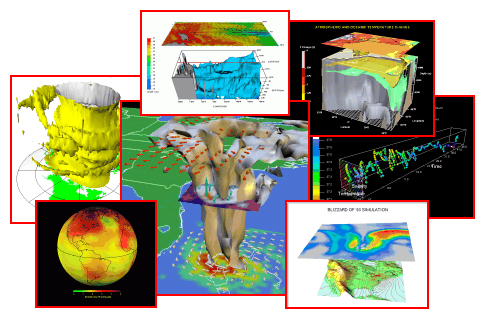## Statistics and Data Analysis

Statistics is a the branch of mathematics that studies collections of data, providing a way of analysing it, interpretating it and presentating it. While Charlie uses its techniques in a crime scene investigation, it is actually used in a wide variety of scientific or science-related fields.

One of the most basic uses of statistical methods is to provide people like Charlie with means of summarizing and describing large or complex sets of data; this is called descriptive statistics. In more advanced situations, patterns in the data may be even modeled so as to account for randomness in the observations, and then used to draw inferences about the process or population being studied; this is called inferential statistics.

We will focus in this lesson on the first kind of uses, and we will explore a few ways of summarizing large collections of data, starting with the most obvious ones.

### Statistics 101

The fundamental ingredient in data analysis is obviously the data itself. In this episode Charlie is trying to investigate various properties of several fire cases in order to establish what he calls a "fireprint". For each given fire case, he has access to informations about the fire such as the rate of burn, the length of scorch marks and the amount of gasoline remnants, for example. In order to make this data comprehensible, he needs to extract a "fireprint", i.e. the most important features out of those hundreds of sample.

Activity 1 Suppose we are looking at the following table, where each line is a sample representing a fire case.

 Length of scorch marks l Rate of burn r 1.0 4.3 1.2 4.5 2.2 4.9 1.4 5.6 1.4 3.9 1.1 4.1 1.8 3.9 1.9 4.9 2.1 5.0

We will investigate some basic operations that can still extract valuable information from a given collection.

#### Mean or average

Given a collection of samples x1, ..., xn, one can forme the mean of the samples using by taking the average of all the xi's. That is to say, mean =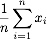.

1. What is the mean length of scorch marks? the mean rate of burn?
2. What is the mean value of the quantity (length of scorch marks + 3 * rate of burn)? Can you find it directly using the previous question?
3. Plot the points (l, r) in the x,y-plane and place the point whose coordinates are the mean l and r values respectively. Do you notice anything? What is the barycenter of the collection of points?

#### Median

The mean of a collection of samples by itself doesn't always reflect the right notion of "average" sample value. The mean salary of a baseball player in the majors leagues, for example is \$1.2 million dollars. Only given this fact, it is easy to see how the perception of the overpaid baseball player was created.

However, it is often conveniently ignored that the median salary for major leaguers is only \$410,000, where the median is the "middle" salary for baseball players: 50% make more than \$410,000, but 50% make less.

1. What is the median of the following collection: 5, 6, 7, 15, 20?
2. What should be the median in the following case: 2, 5, 10, 15? Should it be 5, 10 or 7.5? Discuss the different possibilities.

#### Variance

Another important source of information is provided by the variance of our collection. While the mean and the median provide a sense of what the "middle" value is, they do not reflect how spread the data is. For example, the following collections have the same mean and median: {-1, 0, 1} and {-100, 0, 100}. The variance is there to fill in this gap. Here is how it is calculated: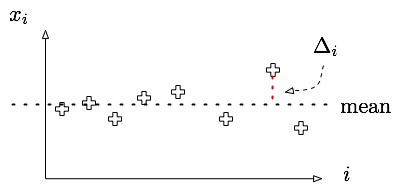First, calculate the mean of the samples. Then, for each sample, find the distance between the mean and the sample. Finally, take the average of the square of that distance by summing over all samples, and dividing by the number of samples
1. Write an equation for the variance of a collection, following the given instructions
2. Calculate the variance in the rate of burn from the table above
3. Consider now the squared rate of burn. Compute the variance of this new variable, and call it A.
4. Compute the mean rate of burn and call it B
5. Now evaluate A - B2 and compare your answer with that of question 2
6. The above equation is in fact no coincidence and can be shown to be true in general. Consider a collection X of samples {x1, ..., xn}, and the collection X2 with samples {x12, ..., xn2}. Compare Mean(X2) - Mean(X)2 and Variance(X).

#### Covariance

Suppose now you are given a collection of samples (xi, yi) describing the distribution of height and weight in a classroom. One might wonder whether there is any relationship between those two variables, meaning how accurate is it to guess someone's weight knowing their height, for example.

One measure of such a relationship between two variables can be found by computing the covariance and the correlation factor of the two variables. If xbar and ybar are the mean x and y values respectively, then the covariance and the correlation factor are given by: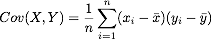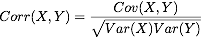1. With have the following pairs of x,y-samples {(1, 2), (2, 3), (4, 10)}. What is the covariance in this case? What about the correlation factor?
2. Plot those points on the x,y-plane and notice that they almost all lie on a single line. What is the sign of the slope of that line? Compare that to the sign of the correlation factor
3. Do the same thing with {(1, 2), (2, 2), (4, 3)} and {(1, 2), (2, -5), (4, -7)}. What do you notice?
4. Can you guess the sign of the correlation factor in the following cases just by looking at the (x,y) plots?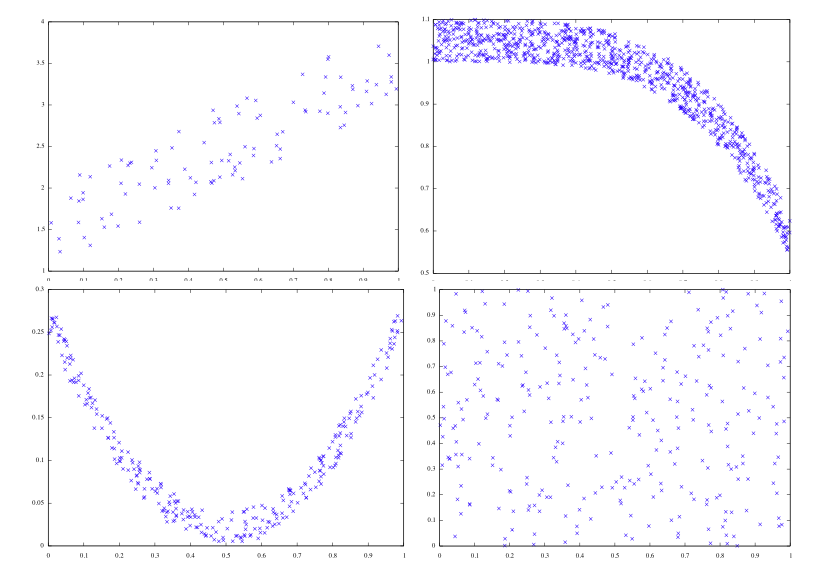The higher the correlation function, the more linear relationship there is between the two variables. Covariance and correlation have to be taken with a grain of salt as their meaning is much more subtle than first appears.

This webpage does a really good job showing that correlation and coveriance do not tell the full story, and thus one should be careful drawing conclusions solely based on their values.

Wikipedia is always a good source of information, and in this case, provides very interesting plots that coulp help understand these two notions.

## Linear Regression

Linear regression is a very widely used technique whose purpose is to find linear trends in large chunks of data. It is used to find the line that best approximates a collection of numerical data points (x1, y1), ..., (xn, yn). Doing that, its reduces the complexity of the data into 2 numerical parameters that specify the approximating line: something like y = 2 x + 3. It can also be used to predict an y-value given an x-value that is not in the samples. This approximating line is also called least squares line.

Suppose we have a collection of a thousand pairs of fire properites, such as the average length of scorch marks and the amount of gasoline remnants, and we would like to reduce this collection of data to 2 parameters by finding the best approximating line in the following sense: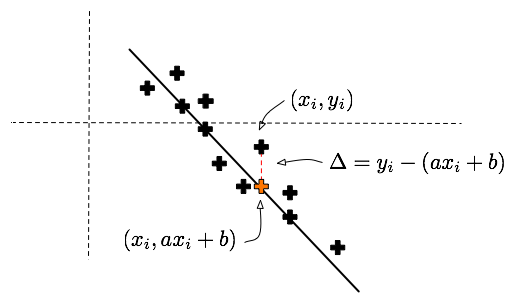Given a line y = a x + b, and a sample (xi, yi), we can calculate the vertical error between that point and the line. This error is given by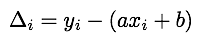If we sum the squares of these errors over all the samples, we get a function of a and b that encodes how far a given line is from being a good approximation to the sample points. The smaller E(a,b), the better the approximation is.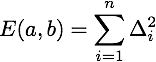In the next activity, we investigate how to find the values of a and b that realize the minimal error.

Activity 2 The locate the minimum of E, we use the gradient technique. Recall that for a one variable function f(x), the minima and maxima are amongst the x's that satisfy f'(x) = 0. This technique actually generalizes very well to functions of several variables.
1. Find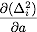and, then find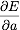and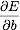2. The minimum of E is obtained when bothandare zero. Show that this is equivalent to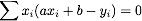and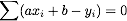3. Finally, solve for a and b, and show that the regression line is parametrized byIn the following application, we explore some examples and applications of this technique.

Activity 3
1. What is the least square line for the points (0, b) and (1, a)? Are you surprised?
2. Do it now for (-1, 2), (0, 1) and (3, -4), and predict the value that would correspond to x = 4.

Activity: Craters of Mars One theory of crater formation suggests that the frequency of large craters should fall off as the square of the diameter (Marcus, Science, June 21, 1968). Pictures from Mariner IV show the frequencies listed in the following table.
 Diameter D in km Frequency F 32-45 51 45-64 22 64-90 14 90-128 4
1. Plot the data points (D, F). Is using a line reasonable in this case?
2. What if we look at 1/D2 rather than D? Use the following table and plot (1/D2, F) using the left value for D in each interval:
 1/D2 Frequency F 0.001 51 0.0005 22 0.00024 14 0.000123 4

Is a line a reasonable approximation now? Find the least mean squares line and write F = a * (1/D)2 + b